TitleCollege Algebra
Answer/Discussion to Practice Problems
Tutorial 18: Solving Polynomial Equations by Factoring

WTAMU > Virtual Math Lab > College Algebra > Tutorial 18: Solving Polynomial Equations by FactoringAnswer/Discussion to 1a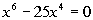Step 1: Simplify each side if needed.

 This polynomial equation is already simplified.

 Step 2: Write in standard form,, if needed.

 This polynomial equation is already in standard form.

 Step 3: Factor.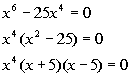*Poly. eq. in standard form *Factor out the GCF *Factor a  diff. of squares

 Step 4:  Use the Zero-Product Principle AND Step 5: Solve for the equation(s) set up in step 4.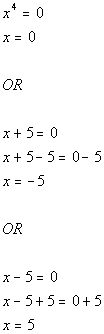*Use Zero-Product Principle *Set 1st factor = 0 and solve       *Set 2nd factor = 0 and solve           *Set 3rd factor = 0 and solve

 There are two solutions to this polynomial equation: x = 0, x = -5 and x = 5.Answer/Discussion to 1bStep 1: Simplify each side if needed.

 This polynomial equation is already simplified.

 Step 2: Write in standard form,, if needed.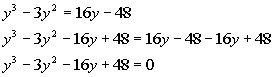*Inverse of add. 16y and sub. 48 is sub. 16y and add. 48 *Poly. eq. in standard form

 Step 3: Factor.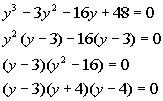*Poly. eq. in standard form *Factor by grouping

 Step 4:  Use the Zero-Product Principle AND Step 5: Solve for the equation(s) set up in step 4.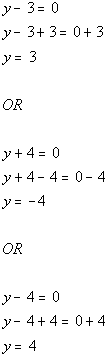*Use Zero-Product Principle *Set 1st factor = 0 and solve         *Set 2nd factor = 0 and solve             *Set 3rd factor = 0 and solve

 There are two solutions to this polynomial equation: y = 3, y = -4 and y = 4.

WTAMU > Virtual Math Lab > College Algebra > Tutorial 18: Solving Polynomial Equations by Factoring

Last revised on Dec. 16, 2009 by Kim Seward.
All contents copyright (C) 2002 - 2010, WTAMU and Kim Seward. All rights reserved.